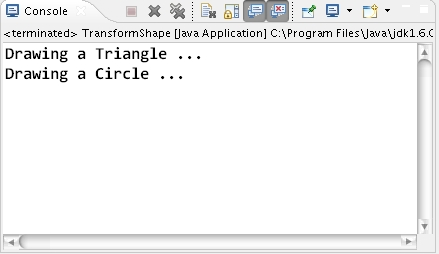A simple demonstration of State Design Pattern in Java through a program

 Tweet Introduction:  So what is a State Design Pattern in Java ? A State Design Pattern is a simple design pattern in java which provides the designer dynamic flexibility at run time. Thus below is the simple Java program that demonstrate the State Design Pattern in java. Here the inheritance shows difference in behavior of the classes and the fields shows the variation in the state of classes. Video tutorial to demonstrate how to implement State Design Pattern in Java. Program to demonstrate State Design Pattern in Java through a program class Shape { public void draw() { } } class Triangle extends Shape { public void draw() { System.out.println("Drawing a Triangle ... "); } } class Circle extends Shape { public void draw() { System.out.println("Drawing a Circle ... "); } } class Drawing { private Shape s = new Triangle(); public void changeShape() { s = new Circle(); } public void startDrawing() { s.draw(); } } public class TransformShape { public static void main(String[] args) { Drawing drawing = new Drawing(); drawing.startDrawing(); drawing.changeShape(); drawing.startDrawing(); } } Output of the program :Introduction:
So what is a State Design Pattern in Java ?
A State Design Pattern is a simple design pattern in java which provides the designer dynamic flexibility at run time. Thus below is the simple Java program that demonstrate the State Design Pattern in java. Here the inheritance shows difference in behavior of the classes and the fields shows the variation in the state of classes.

Video tutorial to demonstrate how to implement State Design Pattern in Java.

Program to demonstrate State Design Pattern in Java through a program

class Shape
{
public void draw()
{

}
}

class Triangle extends Shape
{
public void draw()
{
System.out.println("Drawing a Triangle ... ");
}
}

class Circle extends Shape
{
public void draw()
{
System.out.println("Drawing a Circle ... ");
}
}

class Drawing
{
private Shape s = new Triangle();

public void changeShape()
{
s = new Circle();
}

public void startDrawing()
{
s.draw();
}
}

public class TransformShape {

public static void main(String[] args)
{
Drawing drawing = new Drawing();

drawing.startDrawing();
drawing.changeShape();
drawing.startDrawing();
}

}

Output of the program :

Please Give Us Your 1 Minute In Sharing This Post!
SOCIALIZE IT →